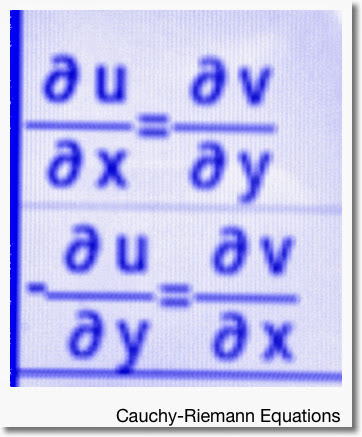## Saturday, November 22, 2014

### Complex Analysis: Cauchy-Reimann EquationsContinuing my blogging adventures at the Last Drop Cafe in Claremont, CA:

Cauchy-Reimann Equations

Let the complex-valued function f(z), where z = x+i*y, be defined in the parametric form:

f(z) = u(x,y) + i*v(x,y) where u(x,y) = Re(f(z)) and v(x,y) = Im(f(z)).

Then by the definition of the derivative:

f(z) = lim x0 → x [(f(z) + x0) - f(z))/(x - x0)]
= lim x0 → x [(u(x +x0, y) - u(x, y) + i*v(x + x0, y) - i*v(x, y))/(x - x0)]
= du/dx + i*dv/dx (I)

Also:

f(z) = lim y0 → y [(f(z + y0) - f(z))/(i*y - i*y0)]
= lim y0 → y [(u(x, y + y0) - u(x, y) + i*v(x, y + y0) - i*v(x, y))/(i*(y - y0))]
= -i*(lim y0 → y [(u(x, y + y0) - u(x, y) + i*v(x, y + y0) - i*v(x, y))/(y - y0)])
= -i*du/dy + dv/dy (II)

Taking the real and imaginary parts of (I) and (II):

du/dx = dv/dy and -du/dy = dv/dx

The Cauchy-Riemann equations can be used to determine whether f(z) is differentiable and if so, where.

Two Quick Examples

1. z^2 = (x^2 - y^2) + 2*i*x*y

u=x^2 - y^2
v=2*i*x*y

du/dx = 2*x, dv/dy = 2*x
-du/dy = 2*y, dv/dx = 2*y (note the negative sign in front of du/dy!)

Since the Cauchy-Riemann equations hold, and without restriction, then z^2 is differentiable for all z. And:

df/dz = 2*x + 2*i*y = 2*(x+i*y) = 2*z

2. e^z = e^(x+i*y) = e^x*cos y + i*e^x*sin y

u=e^x*cos y
v=e^x*sin y

du/dx = e^x*cos y, dv/dy = e^x*cos y
-du/dy = e^x*sin y, dv/dx = e^x*sin y

Since the Cauchy-Riemann equations hold, and without restriction, then e^z is differentiable for all z. And:

df/dx = e^x*cos y + i*e^x*sin y = e^z

I used (I), but using (II) will garner the same result.

That is the Cauchy-Riemann equations in a nutshell!

Source: Wunsch, A. David. Complex Variables with Applications. 2nd Edition. Addison-Wesley Publishing Company. 1994.

As always, have a great day! Take care. Eddie

This blog is property of Edward Shore. 2014